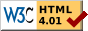## Dokumentation zu: xor_bits(E)``` SYNOPSIS string xor_bits(string str1, string str2) DESCRIPTION and are both bitstrings. The result of the function is a bitstring with the binary-xor of and , ie. a string in which a bit is set only if the corresponding bits in either or (but not both) is set. EXAMPLES string s1, s2, s3; s1 = set_bit("", 3); s1 = set_bit(s1, 15); -> s1 is "( (" s2 = set_bit("", 3); s2 = set_bit(s2, 4); -> s2 is "8" s3 = xor_bits(s1, s2); -> s3 is now "0 (", ie. a bitstring with bits 4 and 15 set. SEE ALSO clear_bit(E), set_bit(E), test_bit(E), next_bit(E), last_bit(E), count_bits(E), and_bits(E), or_bits(E), invert_bits(E), copy_bits(E) ``` Die Seite ist auch in Deutsch vorhanden.

Start » Magierhandbuch » Docu » Efun » Xor_bits Letzte Generierung: 25.04.2021, 01:58mud@wl.mud.de